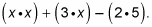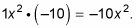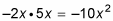##### Trigonometry Workbook For DummiesFor polynomials with a nonprime leading coefficient and constant term, you can use a procedure called the FOIL method of factoring (sometimes called the British Method). The FOIL Method always works for factoring trinomials and is a very helpful tool if you can’t wrap your brain around guess-and-check. When the FOIL method fails, you know for certain the given quadratic is prime.

The FOIL method of factoring calls for you to follow the steps required to FOIL binomials, only backward. Remember that when you FOIL, you multiply the first, outside, inside, and last terms together. Then you combine any like terms, which usually come from the multiplication of the outside and inside terms.

For example, to factor x2 + 3x – 10, follow these steps:

1. Check for the Greatest Common Factor (GCF) first.

The expression x2 + 3x – 10 doesn’t have a GCF when you break it down and look at it. The breakdown looks like this:No factors are common to all terms, so the expression has no GCF. You get to move on to the next step.

2. Multiply the quadratic term and the constant term.

Be careful of the signs when you do this step. In this example, the quadratic term is 1x2 and the constant is –10, hence3. Write down all the factors of the result that result in pairs in which each term in each pair has an x.

The pairs factors of –10x2 in which each term contains an x are –1x and 10x, 1x and –10x, –2x and 5x, and 2x and –5x.

4. From this list, find the pair that adds to produce the coefficient of the linear term.

You want the pair whose sum is +3x. For this problem, the answer is –2x and 5x becauseand –2x + 5x = 3x.

5. Break up the linear term into two terms, using the numbers from Step 4 as the coefficients.

Written out, you now have x2 – 2x + 5x – 10.

Life is easier in the long run if you always arrange the linear term with the smallest coefficient first. That’s why we put the –2x in front of the +5x.

1. Group the four terms into two sets of two.

Always put a plus sign between the two sets: (x2 – 2x) + (5x – 10).

2. Find the GCF for each set and factor it out.

What do the first two terms have in common? An x. If you factor out the x, you have x(x – 2). Now, look at the second two terms. They share a 5. If you factor out the 5, you have 5(x – 2). The polynomial is now written as x(x – 2) + 5(x – 2).

3. Find the GCF of the two new terms.

As you can see, (x – 2) appears in both terms, so it’s a GCF. Factor out the GCF from both terms (it’s always the expression inside the parentheses) to the front and leave the remaining terms inside the parentheses. Thus x(x – 2) + 5(x – 2) becomes (x – 2)(x + 5). The (x + 5) is the leftover after factoring out the GCF of (x-2).

Sometimes the sign has to change in Step 6 in order to correctly factor out the GCF. But if you don’t start off with a plus sign between the two sets, you may lose a negative sign you need to factor all the way. For example, in factoring x2 – 13x + 36, you end up in Step 5 with the following polynomial: x2 – 9x – 4x + 36. When you group the terms, you get (x2 – 9x) + (–4x + 36). Factor out the x in the first set and the 4 in the second set to get x(x – 9) + 4(–x + 9). Notice that the second set is the exact opposite of the first one? In order for you to move to the next step, the sets have to match exactly. To fix this, change the +4 in the middle to –4 and get x(x – 9) – 4(x – 9). Now that they match, you can factor again.

Even when an expression has a leading coefficient besides 1, the FOIL method still works. The monkey wrench comes only if in Step 2 you can’t find any factors that add to give you the linear coefficient. In this case, the expression is prime. For example, in 2x2 + 13x + 4, when you multiply the quadratic term of 2x2 and the constant of 4, you get 8x2. However, no factors of 8x2 also add to be 13x, so 2x2 + 13x + 4 is prime.